## Order States in Linearized Model

### Control State Order of Linearized Model Using Model Linearizer

This example shows how to control the order of the states in your linearized model. This state order appears in linearization results.

1. Open and configure the model for linearization by specifying linearization I/Os and an operating point for linearization. You can perform this step as shown, for example, in Linearize at Trimmed Operating Point. To preconfigure the model at the command line, use the following commands.

```sys = 'magball'; open_system(sys) sys_io(1) = linio('magball/Controller',1,'input'); sys_io(2) = linio('magball/Magnetic Ball Plant',1,'openoutput'); setlinio(sys,sys_io) opspec = operspec(sys); op = findop(sys,opspec);```

These commands specify linear analysis points at the input and output of the plant and compute the steady-state operating point.

2. Open the Model Linearizer for the model.

In the Simulink® model window, in the Apps gallery, click Model Linearizer.

3. Open the Options for exact linearization dialog box.

On the Linear Analysis tab, click.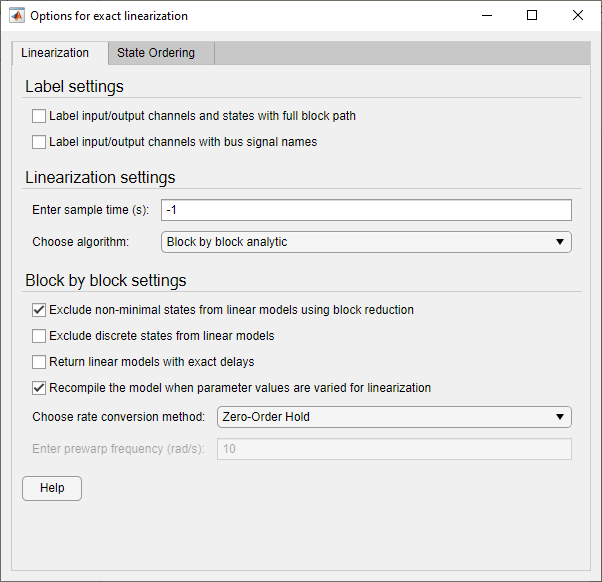4. In the dialog box, on the State Ordering tab, select Enable state ordering.

5. Specify the desired state order using the and buttons.

Tip

If you change the model while Model Linearizer is open, click to update the list of states.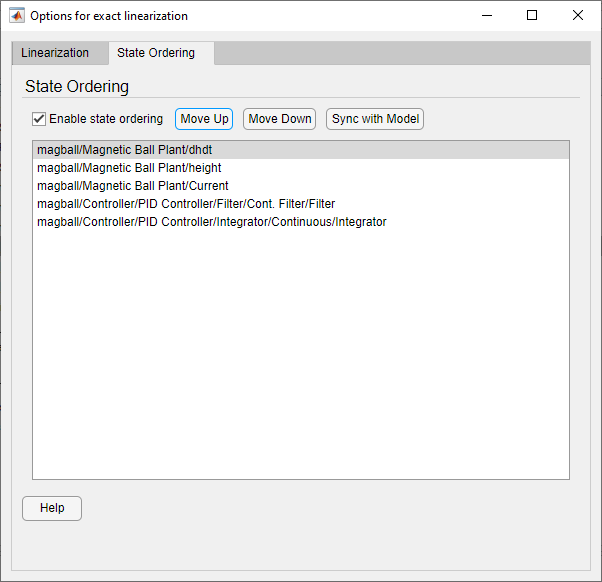Close the dialog box.

6. Enable the linearization result viewer. On the Linear Analysis tab, select Result Viewer.When this option is selected, the result viewer appears when you linearize the model, enabling you to view and confirm the state ordering.

Tip

If you do not check Result Viewer, or if you close the result viewer, you can open the result viewer for a previously linearized model. To do so, in the Plots and Results tab, select the linear model in the Linear Analysis Workspace, and click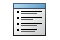Result Viewer.

7. Linearize the model. For example, click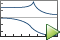Bode.

A new linearized model, `linsys1`, appears in the Linear Analysis Workspace. The linearization result viewer opens, displaying information about that model.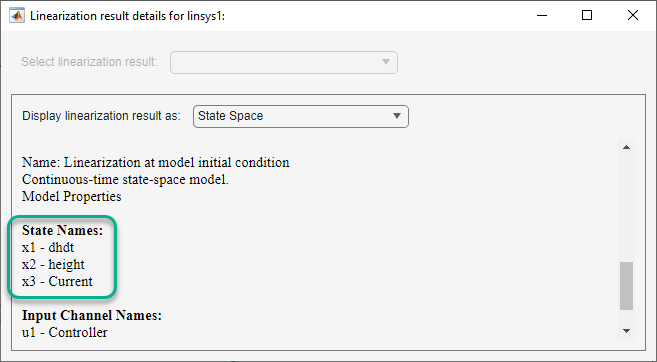The linear model states appear in the specified order.

### Control State Order of Linearized Model Using MATLAB Code

This example shows how to control the order of the states in your linearized model. This state order appears in linearization results.

1. Load and configure the model for linearization.

```sys = 'magball'; load_system(sys); sys_io(1)=linio('magball/Controller',1,'input'); sys_io(2)=linio('magball/Magnetic Ball Plant',1,'openoutput'); opspec = operspec(sys); op = findop(sys,opspec);```

These commands specify the plant linearization and compute the steady-state operating point.

2. Linearize the model, and show the linear model states.

```linsys = linearize(sys,sys_io); linsys.StateName```

The linear model states are in default order. The linear model includes only the states in the linearized blocks, and not the states of the full model.

```ans = 'height' 'Current' 'dhdt' ```
3. Define a different state order.

```stateorder = {'magball/Magnetic Ball Plant/height';... 'magball/Magnetic Ball Plant/dhdt';... 'magball/Magnetic Ball Plant/Current'};```
4. Linearize the model again and show the linear model states.

```linsys = linearize(sys,sys_io,'StateOrder',stateorder); linsys.StateName```

The linear model states are now in the specified order.

```ans = 'height' 'dhdt' 'Current'```